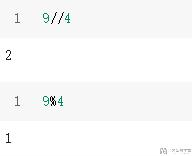# Python入门教程–基础语法2018年2月3日19:46:21

2 2859字阅读9分31秒

## 数据类型

• int　整形
• float　浮点型
• complex　复数
• None 空值,与０不同

### 数据类型的转换

• int(x) 将x转换为整形
• float()

### 例子

``````#下面代码在ipython中进行测试
a = 1
type(a)
>> int
a = float(a)
type(a)
>> float``````

## python查看内存地址

1. def tt(n, memo={}):
2.     # 终止条件
3.     if n == 1:
4.         memo[n] = 1
5.         return
6.     # 调用递归
8.     tt(n=n-1)
9.     # 处理数据
10.     memo[n] = n
12. if __name__ == "__main__":
13.     tt(n=3)

## python字符串的使用

### `\`的使用

• `\`作为`python`中的转义符

``````a = '\'hello world\''
print(a)
>> 'hello world'``````
• 字符串末尾`\`来进行换行

``````str１ = 'it is a string\
.it is a string.'
print(str１)``````

### 字符串的连接

• 使用`+`来连接字符串
``````a = 1
b = str(a)+'+'+str(a)+'=2' #这里思考为什么要用`str(a)`
print(b)
>> 1+1=2``````

### 字符串切片

``````a = '1234567'
a
>> '1'
a[-1]
>> '7'

#返回字符串长度
len(a)``````

### 字符串切割

``````a = 'hello:world'
a.split(':')
>> ['hello','world']``````

## 运算符

### 数学运算符

`python3`中的数学运算符与数学中的运算符基本类似, 这里介绍一下几个不熟悉的.

1. 9//4
2. >> 2
3. 9%4
4. >> 11. 2**3
2. >> 8

### 逻辑运算符

``````True and False
True or False``````

## 控制结构

### 选择控制

``````a = 10
if a>15:
print('a>5')
elif a>10:
print('15>a>10')
else:
print('a<10')

>> 'a<10'``````

### 循环控制

#### for循环

for循环主要是依次取出列表中的一个项目,对列表进行遍历处理

``````for i in '1234567':#思考为什么这里可以这么写
print(i)

for i in range(7):
print(i)``````

#### while循环

``````a = 10
while a>5:
print(a)
a = a-1``````

#### break和continue

`break`--停止当前循环 `continue`--跳过当前循环中的一轮

``````for a in range(10):
if a==5:
break
print(a)#这里输出到4时停止

for a in range(10):
if a==5:
continue
print(a)#这里输出里没有5``````

## 异常处理

### 使用raise

1. def ThorwErr():
2.     raise Exception("抛出一个异常")
3. ThorwErr()

1. Traceback (most recent call last):
2.   File ".\ra.py", line 4, in <module>
3.     ThorwErr()
4.   File ".\ra.py", line 2, in ThorwErr
5.     raise Exception("抛出一个异常")
6. Exception: 抛出一个异常

### 使用try与except

``````try:
pass#这里可以输入其他一些代码
except:
print('Error')``````

## 注释

`python`中,注释需要以`#`开头

### 例子

``````#下面是注释的例子
print(1+1) #打印1+1的结果

#下面是多行注释
'''
print(1+1)
'''``````

## 练习

``````python3 test.py 7
>> 1*7=7
2*7=14
3*7=21
4*7=28
5*7=35
6*7=42
7*7=49
8*7=56
9*7=63

python3 test.py a
>> 请输入数字``````

### 参考答案

``````import sys

try:
num = int(sys.argv)
except:
print('请输入数字')
exit()

for i in range(1,10):
print(str(i)+'*'+str(num)+'='+str(num*i))``````

~~ by WMN

• 微信公众号
• 关注微信公众号
•• QQ群
• 我们的QQ群号
•• 本文由 发表于 2018年2月3日19:46:21
• 转载请务必保留本文链接：https://mathpretty.com/8938.html